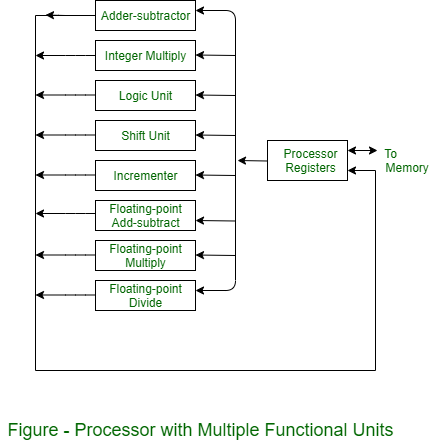Open in App
Not now

# What is Parallel Processing ?

• Difficulty Level : Basic
• Last Updated : 22 Apr, 2020

For the purpose of increasing the computational speed of computer system, the term ‘parallel processing‘ employed to give simultaneous data-processing operations is used to represent a large class. In addition, a parallel processing system is capable of concurrent data processing to achieve faster execution times.

As an example, the next instruction can be read from memory, while an instruction is being executed in ALU. The system can have two or more ALUs and be able to execute two or more instructions at the same time. In addition, two or more processing is also used to speed up computer processing capacity and increases with parallel processing, and with it, the cost of the system increases. But, technological development has reduced hardware costs to the point where parallel processing methods are economically possible.

Parallel processing derives from multiple levels of complexity. It is distinguished between parallel and serial operations by the type of registers used at the lowest level. Shift registers work one bit at a time in a serial fashion, while parallel registers work simultaneously with all bits of simultaneously with all bits of the word. At high levels of complexity, parallel processing derives from having a plurality of functional units that perform separate or similar operations simultaneously. By distributing data among several functional units, parallel processing is installed.

As an example, arithmetic, shift and logic operations can be divided into three units and operations are transformed into a teach unit under the supervision of a control unit.

One possible method of dividing the execution unit into eight functional units operating in parallel is shown in figure. Depending on the operation specified by the instruction, operands in the registers are transferred to one of the units, associated with the operands. In each functional unit, the operation performed is denoted in each block of the diagram. The arithmetic operations with integer numbers are performed by the adder and integer multiplier.

Floating-point operations can be divided into three circuits operating in parallel. Logic, shift, and increment operations are performed concurrently on different data. All units are independent of each other, therefore one number is shifted while another number is being incremented. Generally, a multi-functional organization is associated with a complex control unit to coordinate all the activities between the several components.The main advantage of parallel processing is that it provides better utilization of system resources by increasing resource multiplicity which overall system throughput.

My Personal Notes arrow_drop_up